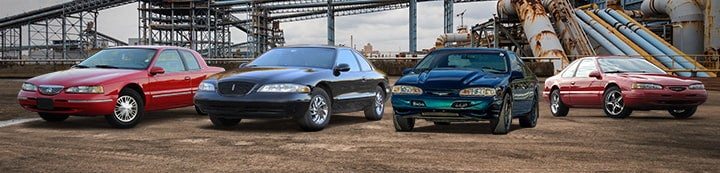1 - 4 of 4 Posts

#### AverageJoe

·
##### Sheepish
Joined
·
4,085 Posts
Discussion Starter
This could be an interesting technical debate.

Why do the hp and tq curves seem to ALWAYS cross at 5200 rpms? Does anybody know?#### Matt S.

·
##### Registered
Joined
·
620 Posts
Now I don't know much about the subject, but isn't that the conversion factor to get hp from rpm and torque values. I think the equation is (hp)=(rpm*torque/5225) So setting hp=torque you get rpm=5225. Therefore where the hp and torque curves meet should always be at about 5200 rpms. Hope that's it????

#### jturmel

·
##### Registered
Joined
·
1,848 Posts
Okay here's the short explanation.

First understand there is a difference between ft-lb and lb-ft, ft-lb is the is used to describe work, while lb-ft is used to describe torque. Work being once something has been done, you can have torque on an object without anything actually being accomplished.

Basically when the expression "horsepower" came into play, by James Watts, he observed one hp as the amount of power it took to move (perform) 33,000 ft-lb in one minute. One lb-ft of torque for one revolution is equal to 6.2832 ft-lb of work actually being done. (2 * Pi * r)

The formula for HP is as follows:

HP = (torque x rpm x 6.2832) / 33,000

when you simply this formula you get:

HP = (torque x rpm) / 5252

(you're just dividing the top by 6.2832 and the bottom by 6.2832.)

Since dividing a number by itself always results in 1 then that is why they always cross at 5252. (Inserting 5252 into the "rpm" variable in the equation.)

Hope this makes sense.

-J

#### tbirdbrain

·
Joined
·
2,725 Posts
My professors cap is onkudos for the post okcbird, you are correct.

a few useless facts, just for the sake of it,

the non-rounded value of exerted force of 1 hp. is 32580 ft-lb per minute.

the hp rating refers to the ability to ‘raise’ a weight, my favorites are
to raise 33 pounds 1000’ or 1000 pounds 33’ in 1 minute.

A+ for everyone

professors cap is now off for the night.

1 - 4 of 4 Posts